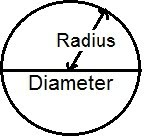# Circle Calculator

Calculate the Area, Diameter, Circumference and Sector area of circle for given values.

Diameter of Circle: [ 2r ]

 Enter the radius : Diameter of Circle :

Area of Circle: [πr² ]

 Enter the radius : Area of Circle :

Circumference of Circle:[ 2πr ]

 Enter the radius : Circumference of Circle :

Area of Sector: [ ½×arc×(angle in degree) ]

 Enter the radius : Enter the angle : Result Area :

Computes the Diameter, Area of Circle, Circumference of Circle and Area of Sector for given values.

The locus of points equidistant from a given point is a circle or we can say the circle is the set of all points equidistant from a central point.

The distance around the edge of the circle is called Circumference.

The distance from the center to the edge is called Radius.

Diameter starts at one side of the circle and passes through the center and ends on the other side.```π = pi = 3.1415926535898